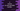# C program to reverse an user provided number## Introduction :

In this tutorial, we will learn how to reverse a number in C. Our program will take one number as input from the user, reverse it and print it out.

With this program, we will learn how to use a while loop, how to read user inputs and how to print out a result.

## C program :

``````#include <stdio.h>

int main()
{
//1
int number;
int reverse = 0;
int rem;

//2
printf("Enter a number: ");
scanf("%d", &number);

//3
while (number != 0)
{
rem = number % 10;
reverse = reverse * 10 + rem;
number /= 10;
}

//4
printf("The reversed number is = %d\n", reverse);

return 0;
}``````

## Explanation :

The commented numbers in the above program denote the step numbers below :

1. Create three variables number, reverse and rem. Variable number is to read and store the user input number, reverse is to store the reversed number and rem is to store the remainder.
2. Ask the user to enter a number. Read it and store it in the number variable.
3. Using a while loop, generate the reversed number. On each step of the while loop, we are taking the rightmost digit of the number and appending it to the right of the reversed number. Once the while loop is finished, the reverse number will be generated.
4. Finally, print out the result to the user.

## Sample Output :

``````Enter a number: 12345
The reversed number is = 54321

Enter a number: 78912
The reversed number is = 21987

Enter a number: 998976
The reversed number is = 679899``````